# Important Questions For Class 11 Maths Chapter 3 - Trigonometric Functions

Important Questions For Class 11 Maths Chapter 3 Trigonometric Functions are provided at BYJU’S to help the students with their examination preparation for the board exams of 2022-2023. Students can go through with these important questions of Trigonometric Functions which are given based on the new pattern prescribed by CBSE.

In Class 11 Trigonometric Functions is the easiest one among all other chapters. Though there are a number of formulas, students can easily score the marks for this chapter. The chapter contains more weightage. Students can refer to the detailed explanations of all the important questions of this chapter here, along with the practice questions. Also, get important questions for Class 11 Maths for all the chapters at BYJU’S.

## Important Questions & Answers For Class 11 Maths Chapter 3 Trigonometric Functions

Q. No.1: In any triangle ABC, prove that a sin (B – C) + b sin (C – A) + c sin (A – B) = 0.

Solution:

In any triangle ABC,

a/sin A = b/sin B = c/sin C = k

a = k sin A, b = k sin B, c = k sin C

LHS

= a sin (B – C) + b sin (C – A) + c sin (A – B)

= k sin A [sin B cos C – cos B sin C] + k sin B [sin C cos A – cos C sin A] + k sin C [sin A cos B – cos A sin B]

= k sin A sin B cos C – k sin A cos B sin C + k sin B sin C cos A – k sin B cos C sin A + k sin C sin A cos B – k sin C cos A sin B

= 0

= RHS

Hence proved that a sin (B – C) + b sin (C – A) + c sin (A – B) = 0.

Q.No.2: Find the radius of the circle in which a central angle of 60° intercepts an arc of length 37.4 cm (use π = 22/7).

Solution:

Given,

Length of the arc = l = 37.4 cm

Central angle = θ = 60° = 60π/180 radian = π/3 radians

We know that,

r = l/θ

= (37.4) * (π / 3)

= (37.4) / [22 / 7 * 3]

= 35.7 cm

Hence, the radius of the circle is 35.7 cm.

Q. No.3: A wheel makes 360 revolutions in one minute. Through how many radians does it turn in one second?

Solution:

Given,

Number of revolutions made by the wheel in 1 minute = 360

1 minute = 60 seconds

Number of revolutions in 1 second = 360/60 = 6

Angle made in 1 revolution = 360°

Angles made in 6 revolutions = 6 × 360°

Radian measure of the angle in 6 revolutions = 6 × 360 × π/180

= 6 × 2 × π

= 12π

Hence, the wheel turns 12π radians in one second.

Q. No. 4: Find the value of √3 cosec 20° – sec 20°.

Solution:

√3 cosec 20° – sec 20°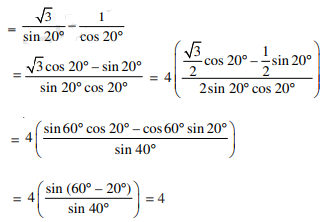Q. No. 5: Show that tan 3x tan 2x tan x = tan 3x – tan 2x – tan x.

Solution:

Let 3x = 2x + x

Taking “tan” on both sides,

tan 3x = tan (2x + x)

We know that,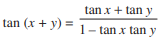tan 3x = (tan 2x + tan x)/ (1- tan 2x tan x)

tan 3x(1 – tan 2x tan x) = tan 2x + tan x

tan 3x – tan 3x tan 2x tan x = tan 2x + tan x

tan 3x – (tan 2x + tan x) = tan 3x tan 2x tan x

Therefore, tan 3x – tan 2x – tan x = tan 3x tan 2x tan x.

Q. No. 6: Prove that: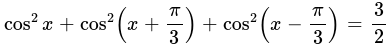Solution:

LHS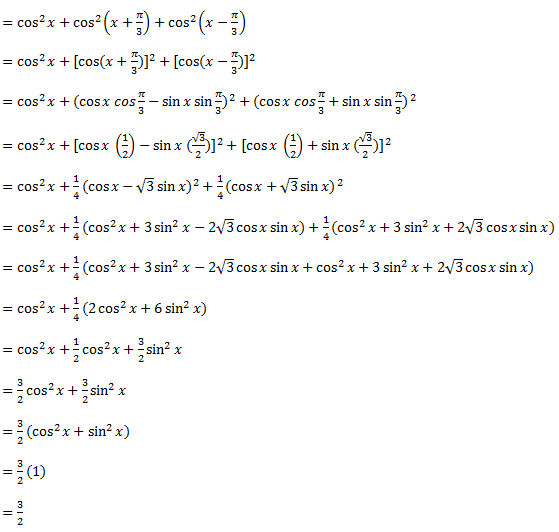= RHS

Hence proved.

Q. No. 7: Find the value of cos 570° sin 510° + sin (-330°) cos (-390°).

Solution:

LHS =cos (570)sin (510) + sin (- 330)cos (- 390)

= cos (570) sin (510) + [ – sin (330) ]cos (390) [ because sin( – x ) = – sin x and cos( – x ) = cos x ]

= cos (570)sin(510) – sin (330)

= cos (90 * 6 + 30) sin (90 * 5 + 60) – sin (90 * 3 + 60) cos (90 * 4 + 30)

= – cos (30) cos (60) – [ – cos (60) ] cos (30)

= – cos (30) cos (60) + cos (30) sin (60)

= 0

Q. No. 8: Find the general solution of the following equation.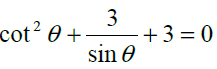Solution:

Given,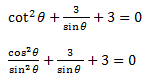cos2θ + 3 sin θ + 3 sin2θ = 0

1 – sin2θ + 3 sin θ + 3 sin2θ = 0

2 sin2θ + 3 sin θ + 1 = 0

2sin2θ + 2 sin θ + sin θ + 1 = 0

2 sin θ (sin θ +1 ) + 1 (sin θ + 1) = 0

(2 sin θ + 1) (sin θ + 1) = 0

2 sin θ + 1 = 0, sin θ + 1 = 0

sin θ = -½, sin θ = -1

θ = nπ – (-1)n π/6, θ = nπ – (-1)n π/2; n ∈ Z

Q. No. 9: Show that 2 sin2β + 4 cos (α + β) sin α sin β + cos 2 (α + β) = cos 2α.

Solution:

LHS = 2 sin2β + 4 cos (α + β) sin α sin β + cos 2(α + β)

= 2 sin2β + 4 (cos α cos β – sin α sin β) sin α sin β + (cos 2α cos 2β – sin 2α sin 2β)

= 2 sin2β + 4 sin α cos α sin β cos β – 4 sin2α sin2β + cos 2α cos 2β – sin 2α sin 2β

= 2 sin2β + sin 2α sin 2β – 4 sin2α sin2β + cos 2α cos 2β – sin 2α sin 2β

= (1 – cos 2β) – (2 sin2α) (2 sin2β) + cos 2α cos 2β

= (1 – cos 2β) – (1 – cos 2α) (1 – cos 2β) + cos 2α cos 2β

= cos 2α

= RHS

Therefore, 2 sin2β + 4 cos (α + β) sin α sin β + cos 2 (α + β) = cos 2α

Q. No. 10: Prove that: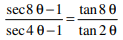Solution:

LHS=(sec 8θ -1 )/ (sec4θ -1)

=(1/(cos8θ) -1 )/ (1/(cos4θ) -1)

=((1-cos8θ)cos4θ )/ ((1-cos4θ) cos8θ)

=(2sin2 4θcos4θ )/ ((2sin2 2θ) cos8θ)

=(2sin4θcos4θsin4θ )/ ((2sin2 2θ) cos8θ)

=(sin8θ * 2sin2θcos2θ )/ ((2sin2 2θ) cos8θ)

=(tan8θ * cos2θ )/ (sin2θ)

= (tan8θ) /(tan 2θ)

= RHS

Hence proved.

### Video Lesson on Trigonometry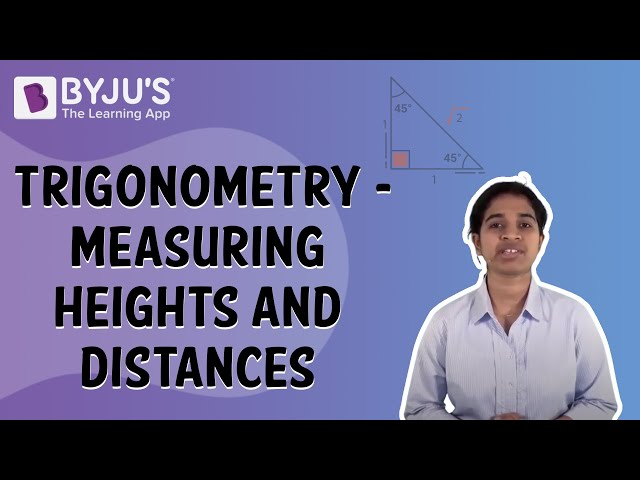### Practice Questions For Class 11 Maths Chapter 3 Trigonometric Functions

1. Find the value of the below expression.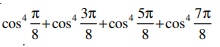Hint: Simplify the expression to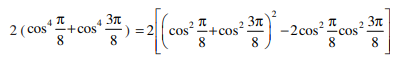2. Find the general solution of the equation 5cos2θ + 7sin2θ – 6 = 0.
3. If θ lies in the first quadrant and cos θ = 8/17, then find the value of cos (30° + θ) + cos (45° – θ) + cos (120° – θ).
4. Prove that: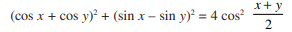5. Prove that cot 4x (sin 5x + sin 3x) = cot x (sin 5x – sin 3x).
6. In triangle ABC, prove that: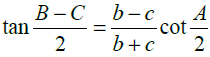7. In any triangle, ABC, show that: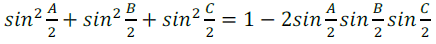8. Find the general solution of the following equation:
tan2θ +(1 – √3) tan θ – √3 = 0
9. Find the value of tan 225° cot 405° + tan 765° cot 675°.
10. If a cos 2θ + b sin 2θ = c has α and β as its roots, then prove that tan α + tan β = 2b/(a + c).
11. If cos + cos = 0 = sin + sin β, then prove that cos 2 + cos 2β = -2 cos (α + ).
12. Find the most general value of θ satisfying the equation tan θ = -1 and cos θ = 1/√2 .
13. The value of cos 10 cos 20 cos 30…………cos 1790 is
(a) 1/√2
(b) 0
(c) 1
(d) -1
For more study material and latest update on CBSE/ICSE/State Board/Competitive exams, keep visiting BYJU’S. Also, download the BYJU’S App for interactive study videos.